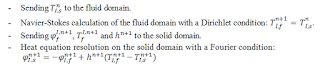Hi,

First thanks for your help and sorry for the time of response,

My problem is the following :

I have a turbine blade in a steady flow, the heat flux and the temperature at the interface are given by a CFD solver called SU2.
So now i need to implement the robin boundary condition. In my case the expression is the following :where the indice I represent the Interface, n the number of iteration, f the fluid field and s the solid field. The h coefficient is calculated at each iteration with the CFD data.
The fourier condition is the other name of the robin boundary condition.

Le vendredi 13 mai 2016 16:53:40 UTC+2, Robert Cimrman a écrit :
This is very similar to the slip (Navier) boundary condition, see the example
. Essentially, just add a boundary integral such as that over Gamma_2
(dw_surface_dot, with the beta coefficient to 1) and then add a Neumann
boundary condition (dw_surface_integrate term(?)). Tell me the exact form of
the Robin condition if you need more help.

r.

 http://sfepy.org/doc-devel/examples/navier_stokes/stokes_slip_bc.html

On 05/13/2016 02:57 PM, Nicolas DELAN wrote:
> Hello,
>
> I work on an aerothermal problem and i need to impose a robin boundary
> condition.
>
> Do anyone know if this boundary condition is implemented in sfepy ?
>
> Thanks,
>